Chapter 20: Optics & Refraction
Author: Paul Riordan-Eva

Optics & Refraction

The correct interpretation of visual information depends on the eye's ability to focus incoming rays of light on the retina. An understanding of this process and how it is influenced by normal variations or ocular disease is essential to the successful use of any optical aid, eg, glasses, contact lenses, intraocular lenses, or low-vision aids. To achieve this understanding, it is necessary to master the concepts of geometric optics, which define the effect on light rays as they pass through different surfaces and media.

GEOMETRIC OPTICS

Speed, Frequency, & Wavelength of Light

Speed, frequency, and wavelength of light are related by the following expression: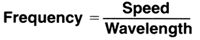In different optical media, speed and wavelength of light change, but frequency is constant. Color depends on frequency, so that the color of a ray of light is not altered as it passes through optical media except by selective nontransmittance or fluorescence. The optical characteristics of a substance can only be defined with respect to clearly specified frequencies of light. A substance to be used for lenses to refract visible light is usually tested with the yellow sodium light (D line) and the blue (F line) and the red (C line) of a rarefied hydrogen discharge tube.

In a vacuum, the speed of all frequencies of light is the same, ie, 299,792.46 kilometers per second (186,282.40 statute miles per second). Since the frequency of the yellow D line is approximately 5.085 × 1014 Hz, the wavelength of this line in a vacuum is 0.5896m. Similarly, the wavelengths in a vacuum of the blue F and red C lines are 0.4861m and 0.6563m, respectively.

Index of Refraction

If the speed of a light ray is altered by a change in the optical medium, refraction of the ray will also occur (Figure 20-1). The effect of an optical substance on the speed of light is expressed as its index of refraction, n. The higher the index, the slower the speed and the greater the effect on refraction.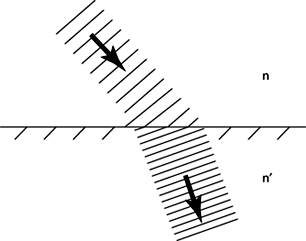Figure 20-1: Refraction of light as it enters a transparent medium of higher refractive index n′

In a vacuum, n has the value of 1.00000. The absolute index of refraction of a substance is the ratio of the speed of light in a vacuum to the speed of light in the substance. The relative index of refraction of a substance is calculated with reference to the speed of light in air. The absolute index of refraction of air varies with the temperature, pressure, and humidity of the air and the frequency of the light, but it is about 1.00032. In optics, n is assumed to be relative to air unless specified as absolute.

Thermal Coefficient of Index of Refraction

The index of refraction changes with the temperature of the medium-it is higher when the substance is colder. This lability of n to temperature is different for different substances. The change in n per degree Celsius for the following substances (all to be multiplied by 10-7) is as follows: glass, 1; fluorite, 10; plastic, 140; water, aqueous, and vitreous, 185. This makes plastic undesirable for precision optical devices. (Plastic also has eight times the thermal expansion of glass.) Water lenses date back to antiquity but are not practical, because of problems with thermal instability, evaporation, freezing, and susceptibility to contamination. It is interesting that in the eye, these objections all but disappear, making the fluid lenses of the eye acceptable.

Dispersion of Light

In a vacuum, the speed of all frequencies of light is the same; thus, the index of refraction is also the same for all colors (1.00000). In all substances, n is different for each color or frequency, being larger at the blue end and smaller at the red end of the spectrum. This difference can be quantified as the dispersion value, V: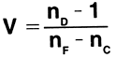where nd, nf, and nc are the indices of refraction for the yellow sodium line and the blue and the red hydrogen lines.

The higher the value of V, the less the dispersion of colors. Table 20-1 gives the indices of refraction and some dispersion values for substances of ophthalmologic interest.

Table 20-1: Indices of refraction and dispersion values of some substances of ophthalmologic interest.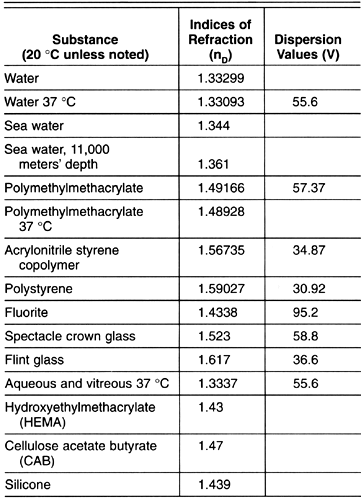Transmittance of Light

Optical materials vary in their transmittance or transparency to different frequencies. Some "transparent" materials such as glass are almost opaque to ultraviolet light. Red glass would be almost opaque to the green frequency. Optical media must be selected according to the specific wavelength of light with which they are to be used.

Laws of Reflection & Refraction

The laws of reflection and refraction were formulated in 1621 by the Dutch astronomer and mathematician Willebrod Snell at the University of Leyden. These laws, together with Fermat's principle, form the basis of applied geometric optics. They can all be stated as follows (Figure 20-2).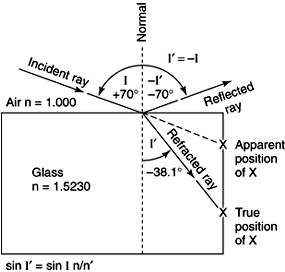Figure 20-2: Example of the laws of reflection and refraction.

1. Incident, reflected, and refracted rays all reside in a plane known as the plane of incidence, which is normal (at a right angle) to the interface.

2. The angle of incidence equals the angle of reflection but has the opposite sign: I = -I′.

3. The product of the index of refraction of the medium of the incident ray and the sine of the angle of incidence of the incident ray is equal to the product of the same terms of the refracted ray. The refracted ray is designated by a prime: n sin I = n′ sin I′ (Snell's law).

4. A ray of light passing from one point to another follows the path that takes the least time to negotiate (Fermat's principle). Optical path length is the index of refraction times the actual path length.

Critical Angle & Total Reflection

In Figure 20-2, consider the ray in the more dense medium as the arriving ray. We see that it is refracted into the less dense medium away from the normal. If we gradually increase the angle of incidence (Figure 20-3) in the denser medium until we reach the critical angle, a startling event takes place: None of the light escapes, but all is suddenly, totally, and perfectly reflected (total internal reflection). This angle is reached as the sine of the incident ray in the denser medium reaches the value -n′/n. This is one method used to determine the index of refraction. For water, with an index of refraction of 1.330, the critical angle has the sine of -1/1.330, or -48.75 degrees.Figure 20-3: Example of the critical angle.

Total reflection obeys the laws of regular reflection, ie, I = - I′. This allows perfect reflection without coatings and is used extensively in prisms and fiberoptics.

In Figure 20-3, the shaded area is not visible from the surface. This is why the angle of the eye cannot be inspected except with the gonioscopic lens (see Chapter 2). The index of refraction of the aqueous-and not the index of refraction of the tears or cornea, as is frequently stated-is the determining factor in this context.

CALCULATIONS USED IN OPTICS

There are two approaches to the application of the principles of geometric optics to single lenses or to compound lens systems. Trigonometric ray tracing is the more valid and exact approach, as it makes no assumptions other than those already determined by the laws of refraction. The algebraic method is a system based on a number of assumptions that greatly simplify calculation of the effects of various lens systems but also limit accuracy to an ever-increasing extent as the lens systems become more complex. The algebraic method cannot be relied on for accurate results, particularly in the assessment of the optical effects of contact lenses, intraocular lenses, and keratorefractive procedures-all of which are becoming more frequently used in the practice of ophthalmology.

Certain considerations are universal to optical calculations whatever method is used. For any optical system, the object and its image are said to lie in conjugate planes. If the object were to be placed in the plane of its own image, the optical system would produce its new image in the original object plane. Thus, the effects of any optical system will be the same for whichever direction light travels through the system. Each optical system has an infinite number of pairs of conjugate planes. Corresponding points on conjugate planes are known as conjugate points.

Trigonometric Ray Tracing

The trigonometric method of ray tracing consists of mathematically plotting the course of certain specified rays through the lens systems. The three rays most frequently traced are shown in Figure 20-4. They are named according to their positions relative to the first refracting surface. The marginal ray enters at the margin of the lens, the paraxial ray very near the optical axis (center of the lens), and the zonal ray in the portion of the lens where the average luminous flux of light passes through the lens. At each refracting surface, the change in direction of each of these rays is calculated according to the principles of Snell's law. This requires knowledge of the radius of curvature of the surface, the index of refraction of the medium on each side of the refracting surface, and the distance to the next surface. Elementary trigonometry is the only mathematical skill necessary for such calculations, though a programmable calculator greatly assists with the number of such calculations that have to be carried out.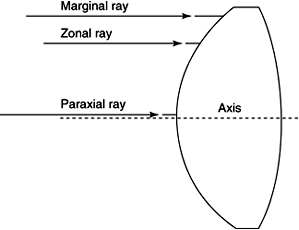Figure 20-4: Illustration of three rays traced in trigonometric ray tracing.

Trigonometric ray tracing provides an exact determination of the point of focus and information on the quality of the image formed by a lens system. The difference between the back focal lengths (distance along the optical axis from the last refracting surface to the point of focus) of the marginal and paraxial rays is a measure of the "spread of focus," thus indicating the degree of spherical aberration (see below). Similarly, if rays of different color (frequency), with their different indices of refraction in each medium, are traced through the system, the degree of chromatic aberration (see below) will be determined. The optical pathway is the sum of the actual distance a ray passes through the substances multiplied by the index of refraction in the various substances through which it passes. How closely the optical pathways of the marginal and paraxial rays match determines the brightness and contrast of the final image.

Trigonometric ray tracing permits determination of the performance of each refracting surface relative to the contribution to the final image. For example, it is easily shown that a planoconvex intraocular lens gives a better image with the convex surface forward and the flat surface closer to the retina. The point of focus often requires-and is easily adjusted by-postoperative refraction. However, the distorted image caused by selecting an intraocular lens of improper shape cannot be repaired by refraction. Suitability in this respect must be achieved by proper preoperative lens design, and this can only be achieved by calculation using the trigonometric method of ray tracing.

Graphic ray tracing is a system comparable to trigonometric ray tracing that uses drawings to determine the optical properties of lens systems; it should not be confused with the method of "ray tracing" described in several books, in which tracings of an image are based on nodal points and focal planes (a derivation of the algebraic method of optical calculations discussed below).

Algebraic Method

Karl Friedrich Gauss (1777-1855) is responsible for refining a method of optical calculations that dispensed with the sines and cosines of the trigonometric method. This assumed that the lenses are "infinitely thin," placed close together, and of small diameter, such that any angle will be so small that the size of the angle measured in radians will have the same value as the sine of the angle and that the sine and the tangent of the angle can be assumed to be the same. The results are the thin lens equations used by opticians to calculate curves for lenses. "Fudge factors" derived from experience are then necessary to correct for the inaccuracies of these equations.

Use of the algebraic method depends on certain definitions. The position of the lens, reduced to a single line, is the principal plane, which intersects the optical axis at the nodal point (optical center). The primary focal point (F) is that point along the optical axis where an object must be placed to form an image at infinity. The secondary focal point (F′) is that point along the optical axis where parallel incident rays are brought to a focus. If the medium on either side of the lens is of the same refractive index, the distance between the nodal point and each of the focal points, the focal length, is the same.

Figure 20-5 shows some of the important thin lens equations.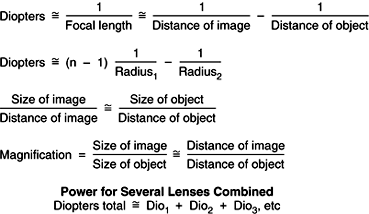Figure 20-5: Algebraic thin lens approximations. All lengths in meters.

The diopter (D) is a measure of lens power derived from the algebraic method of optical calculations. It is defined as the reciprocal of the focal length of a lens in air measured in meters. Diopters are additive, but only for low-power lenses. The result of combining lenses of high power varies greatly with their thickness and the separation distance. High-power lenses must be described by three values: (1) radii of curvature, (2) index of refraction, and (3) thickness.

In Gaussian optics, a thick lens is treated as if there are two nodal points and two principal planes (n and n′ and H and H′ in Figure 20-6). The nodal points lie on the principal planes only if the refractive medium is the same on either side of the lens. The true focal lengths are measured from the principal planes to the focal points, but the front and back focal lengths-essential to the prescription of corrective lenses-are measured from the respective surfaces of the lens to the focal points. The reciprocal of the back focal length corresponds to the back vertex power as measured with a lensometer.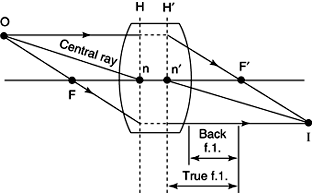Figure 20-6: Description of a thick lens in Gaussian optics.

For making high plus contact lenses or thick spectacle lenses, the equation for dioptric power according to the algebraic method is as follows: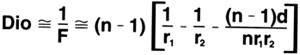where F = focal length, r = radius, and d = thickness of lens, all measured in meters, and n = refractive index.

For contact lenses, a derivation of the thin lens equations is presently used to relate dioptric power to radius of curvature: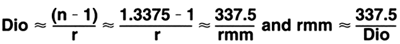n of "cornea" is for this purpose assumed to be 1.3375. rmm = radius in millimeters. These equations are only approximations.

The ray tracing method commonly described in ophthalmic optics texts is a graphic representation of the algebraic system of optical calculations-in comparison to true graphic ray tracing, which is a graphic representation of the trigonometric system. Rays are traced through the optical system to connect conjugate points. The positions of the conjugate planes are derived mathematically from the thin lens equations. The size and orientation of the object are then determined by tracing the central ray, which passes straight through the tip of the image, the nodal point of the lens (without being refracted), and the tip of the object. The rays that traverse the focal points of the lens are derived by extrapolation (Figure 20-7).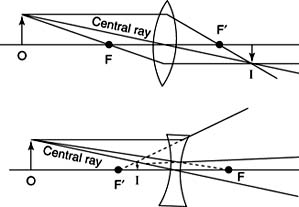Figure 20-7: Ray tracing through plus and minus lenses.

For multiple lens systems, the conjugate planes and the path of the central ray are determined for each lens in succession, producing an image that becomes the object for the next lens until the size and orientation of the final image is located. In the case of a thick lens, refraction occurs at the principal planes of the lens, the position of rays being translated from one principal plane to another without any change in their vertical separation from the optical axis (Figure 20-6). The central ray passes from the tip of the object to the first nodal point and then emerges from the second nodal point parallel to its original direction to reach the tip of the image. When the media on either side of the lens have different refractive indices, the nodal points do not coincide with the principal planes.

Magnification

Linear magnification is the ratio of the height of the image to the height of the object. For an infinitely thin lens in air-as assumed by the algebraic method of optical calculation-this ratio is equal to the ratio of the distance of the image to the distance of the object. For real lens systems, such as those of the eye, a more complex equation including the index of refraction of the initial and final media must be used. Trigonometric ray tracing quickly provides other information necessary for the calculation.

Change of Vertex Distance

If the vertex distance (the distance from the eye) of a lens of given power is altered, the effective power of the lens will also change. To calculate a new lens that will have the same effect at the new distance, a derivation of the thin lens equations can be used: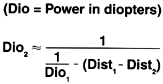Example 1: A + 13 diopter lens at 11mm (0.011 m) is to be replaced by a lens at 9mm (0.009m)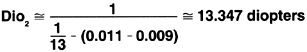Example 2: Same lens to be replaced by a contact lens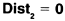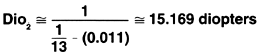This vertex equation is also an approximation and should not be used for intraocular lens calculations, but it is useful for conversion from spectacle to contact lens powers.

Aberrations of Spherical Lenses

Spherical lenses are subject to a number of aberrations that reduce the quality of image produced. The variation of refractive index with frequency of light (dispersion) results in greater refraction of blue than red light (chromatic aberration) (Figure 20-8). Marginal rays are refracted more than paraxial rays, producing spherical aberration (Figure 20-9). Coma, a characteristic comet-shaped blur, is the result of spherical aberration of light originating away from the optical axis of the lens. When light traverses a spherical lens obliquely, there is an additional cylindrical lens effect-astigmatism of oblique incidence. Curvature of field is the production of a curved image from a flat object. Prismatic effects of the lens periphery also cause image distortion. Achromatic lenses may be made by cementing together plus and minus lenses of different refractive indices. The nonchromatic aberrations are overcome by combining or shaping lenses to reduce the power of the lens periphery, by restricting the area of the lens used to the paraxial zones, and by use of meniscus lenses.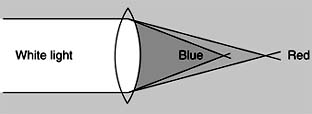Figure 20-8: Chromatic aberration of lenses.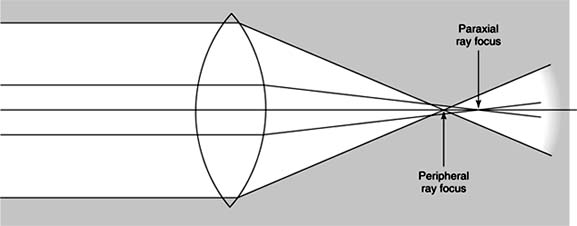Figure 20-9: Spherical aberration of a biconvex lens.

Cylindrical Lenses

A planocylindrical lens (Figure 20-10) has one flat surface and one cylindrical surface, producing a lens with no optical power in the meridian of its axis and maximum power in the meridian 90 degrees away from the axis meridian. The total effect is the formation of a line image, parallel to the axis of the lens, from a point object. The orientation of a planocylindrical lens is specified by the meridian of its axis. The ophthalmic convention for specifying the orientation of the axis of a cylindrical lens is shown in Figure 20-11. Zero begins nasally in the right lens and temporally in the left lens and proceeds in a counterclockwise direction to 180 degrees.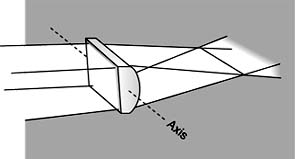Figure 20-10: A planocylindrical lens with axis in the horizontal meridian.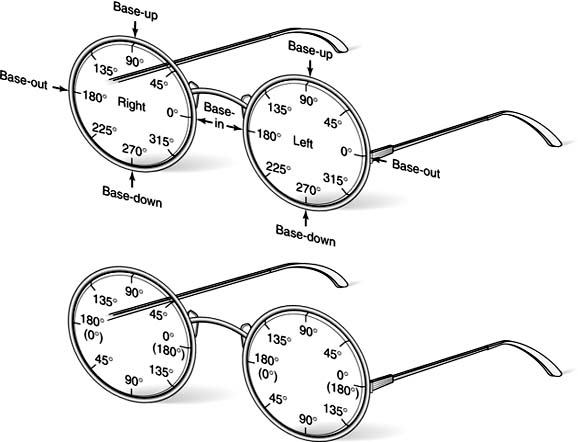Figure 20-11: Top: Illustration of prism base notation. Bottom: Illustration of cylinder axis notation.

In a spherocylindrical lens, the cylindrical surface is curved in two meridians but not to the same extent. In ophthalmic lenses, these principal meridians are at 90 degrees to each other. The effect of a spherocylindrical lens on a point object is to produce a geometric figure known as the conoid of Sturm (Figure 20-12), consisting of two focal lines separated by the interval of Sturm. The position of the focal lines relative to the lens is determined by the power of the two meridians and their orientation by the angle between the meridians. Cross-sections through the conoid of Sturm reveal lines at the focal lines and generally ellipses elsewhere. In one position, the cross-section will be a circle that represents the circle of least confusion.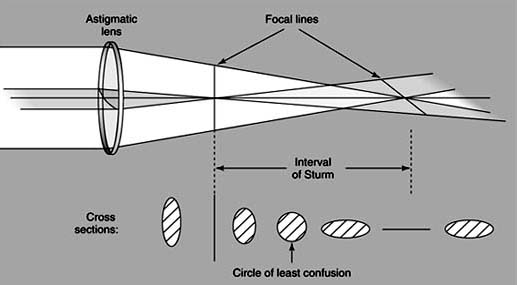Figure 20-12: The conoid of Sturm, formed by light refracted by an astigmatic lens.

A spherocylindrical lens can be thought of as a combination of a spherical lens and a planocylindrical lens. It can then be specified by the orientation of principal meridians and the power acting in each (Figure 20-13). In a cross diagram, the arms are drawn parallel to the principal meridians and labeled with the relevant power. In longhand notation, the cylinder is specified by the orientation of its axis, which is 90 degrees away from the meridian of maximum power.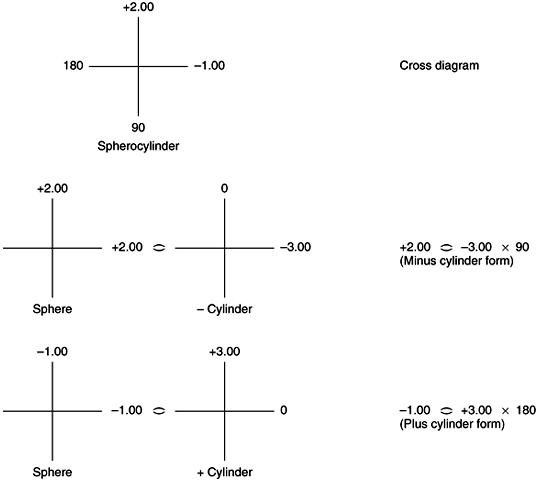Figure 20-13: Cross diagram and equivalent combinations, including longhand notations, for a spherocylindrical lens.

Writing prescriptions for spherocylindrical lenses uses longhand notation, and the lens can be specified in either plus or minus cylinder form (Figure 20-13). The procedure for transposing between these forms is as follows: (1) algebraically sum the original sphere and cylinder; (2) reverse the sign of the cylinder; and (3) change the axis of the cylinder by 90 degrees.

If their principal meridians correspond, combinations of spherocylindrical lenses can be summed mathematically. Otherwise, trigonometric formulas are required. Alternatively, the power of such combinations can be determined by placing them together in a lensometer. The principal meridians of any such combination will be 90 degrees apart.

Prisms

A prism consists of a transparent material with nonparallel flat surfaces. In cross-section, it has an apex and a base. The prism is specified by its power and the orientation of its base.

A prism refracts light toward its base, whereas an object seen through a prism appears deviated toward the apex of the prism. The amount of deviation varies according to the tilt of the prism, ie, the angle of incidence of the light. For glass prisms, calibration is performed in the Prentice position, in which the incident light is perpendicular to the face of the prism (Figure 20-14). For plastic prisms and in general optics, a prism is calibrated in the position of minimum deviation, in which the amount of refraction at the two surfaces of the prisms is equal (Figure 20-14). When prisms are used in clinical practice, these orientations must be adhered to for accurate results.Figure 20-14: Calibration of prisms. Glass prisms and spectacle prisms are calibrated according to the Prentice position, whereas plastic prisms are calibrated according to the position of minimum deviation.

For a glass prism in the Prentice position, the incident ray is not refracted at the first surface because the surfaces are perpendicular to one another (Figure 20-15). At the second surface, the angle of incidence is the same as the apex angle of the prism (A). If I′ is the angle of the final refracted ray, from Snell's law, sin I′ = (n/n′) sin A, n being the refractive index of the prism and n′ the refractive index of the surrounding medium. For example, if the prism is of glass with n = 1.523 and A = 30 degrees, then sin I′ is 1.523 × 0.5, or 0.7615. I′ is 49.6 degrees. The angle of deviation is I′ - A, or 19.6 degrees.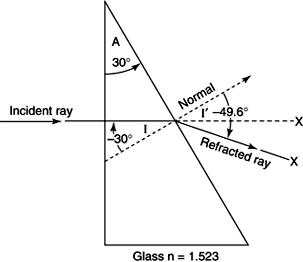Figure 20-15: Example of the prism as used in ophthalmology.

The power of a prism is measured in prism diopters (). One prism diopter deviates an image 1 cm at 1 m (Figure 20-16). The arc tangent of 1/100 is 0.57 degrees. So 1produces an angle of deviation of almost one-half degree. The "rule of thumb" is that a prism of 2produces an angle of deviation of 1 degree, but this cannot be applied to prisms of more than 100.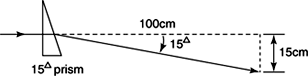Figure 20-16: Power of a prism in prism diopters.

Prisms are used in ophthalmology both to measure and to treat heterotropia and heterophoria. The orientation of a prism's base is indicated by its direction, usually descriptively, ie, "base-up right eye," "base-down left eye," "base-in" or "base-out," or occasionally by a mathematical system (Figure 20-11).

Fresnel prisms are lightweight plastic prisms consisting of narrow, parallel strips of prism with the same apex angle as the desired single prism (Figure 20-17). They are available as press-on prisms for attachment to the back of spectacle lenses, providing an easily adjusted temporary prismatic correction that is less heavy than conventional glass prisms. Their disadvantages are the image degradation due to light scatter and dirt within the grooves.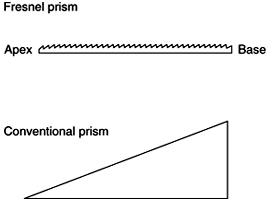Figure 20-17: The Fresnel prism.

Prismatic Effect of Spherical Lenses

Spherical lenses have increasing prismatic power as the light path moves away from the optical center of the lens. The amount of prism power can be calculated from Prentice's rule, which states that the prism power in prism diopters is equal to the dioptric power of the lens in diopters multiplied by the displacement from the optical center in centimeters. For example, at 0.5 cm away from the optical center of a 6+ lens, the prismatic power is 3+. Plus lenses produce prism power with the base oriented toward the optical center of the lens, and minus lenses produce prism power with the base oriented away from their optical center.

The prismatic effect of spherical lenses is an important consideration in the correction of anisometropia. Appropriate spectacle lenses may produce significant vertical prismatic deviation when the peripheral portions of the lenses are used. This occurs mainly when the patient attempts to read. The prismatic effect can be overcome by adopting a chin-down position, thus using the optical centers of the lenses once again, by grinding of a compensatory prism into the reading segment of the glasses (slab-off prism), or by changing to contact lenses.

If a prism needs to be incorporated into a patient's spectacle correction, such as in the control of hypertropia, it may be achieved by decentration of the spherical lens rather than by addition of a prism to the spherical component.

Rapid Detection of Lens Characteristics

The nature of a spherical lens may be rapidly detected by looking through it 0.5 m (19 inches) or so from the eye and moving the lens at right angles to the visual axis. The image seen through a minus (concave) lens will tend to move with the lens. The same test with a plus (convex) lens causes the image to tend to move away from the direction of motion. This effect is due to the prismatic effect of the periphery of the lens. The power of the lens can be approximated by neutralization of these movements by lenses of known power. A cylindrical lens shows changing distortion of the image when the lens is rotated about the visual axis. (Spherical lenses do not.) The orientations of the lens in which the image is clearest indicate the principal meridians. The power in each of the principal meridians can then be determined by the method described above for spherical lenses. A prism is recognized by deviation of the image as the static lens is viewed through its center.

OPTICS & THE EYE

Many attempts have been made to simplify the optical system of the human eye, particularly using the thick lens equations of the algebraic method of optical calculations. Much has been made of the concept that the image on the retina is formed by two lens elements, the cornea contributing about 43 D and the lens the remaining 19 D, but this is a gross oversimplification. The schematic eye of Gullstrand and its reduced form (Figure 20-18) are models from which mathematical values for the optical characteristics of the eye were derived. For instance, in the reduced schematic eye, the cornea is assumed to be the only refracting surface, the principal plane (H) being placed at its apex and a single nodal point (n) at its center of curvature. The globe has an axial length of 22.5 mm, and the refractive index of the eye is said to be 1.33. Unfortunately, these numbers have become accepted by many as true physiologic values rather than as the convenient mathematically derived values they really are. The refractive index of aqueous is about 1.3337 (for the sodium D line at 37 °C).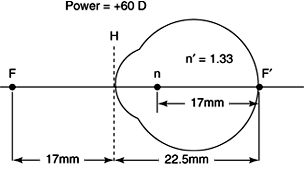Figure 20-18: The reduced schematic eye.

Trigonometric ray tracing demonstrates that the optical system of the human eye is more accurately conceptualized as a three-lens system: the aqueous lens, the lens lens, and the vitreous lens (Figure 20-19). Contrary to popular belief, the cornea itself has almost no power of refraction in the optical system but is important only in shaping the anterior curve of the aqueous lens. The crystalline lens is an interesting optical component because its index of refraction varies throughout its thickness rather than being constant, as assumed in most optical calculations. The vitreous lens is particularly important because of its major effect on magnification.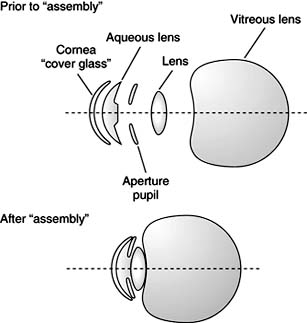Figure 20-19: The optical system of the eye, illustrating the three-lens concept.

Reassessment of models for the optical system of the human eye is essential now that much of ophthalmic surgery, whether it be cataract surgery, keratorefractive procedures, or vitreous surgery, produces profound effects on individual components of the system. Gullstrand's models, in which the system is assumed to function as an integrated unit, cannot be applied under such circumstances.

Accommodation

The eye changes refractive power to focus on near objects by a process called accommodation. Study of Purkinje images, which are reflections from various optical surfaces in the eye, has shown that accommodation results from changes in the crystalline lens. Contraction of the ciliary muscle results in thickening and increased curvature of the lens, probably due to relaxation of the lens capsule.

Visual Acuity

Assessment of visual acuity with the Snellen chart is described in Chapter 2. The average resolving power of the normal human eye is 1 minute of arc. Since the Snellen letters are made from squares of 5 × 5 units (Figure 20-20), the 20/20 size letter has a visual angle of 5 minutes of arc at 20 feet. This is equivalent to 8.7 mm (0.35 inch) width and height. The eye minifies an image at 20 feet by about 350 times. Therefore, the size of the 20/20 letter on the retina is 0.025 mm high and wide. This is equivalent to a resolution capacity of 100 lines per millimeter. For a 6-mm pupil and light of wavelength 0.56m (in air), the absolute theoretic limit would be 345 lines per millimeter.Figure 20-20: Snellen block E.

REFRACTIVE ERRORS

Emmetropia is absence of refractive error and ametropia is the presence of refractive error.

Presbyopia

The loss of accommodation that comes with aging to all people is called presbyopia (Table 20-2). A person with emmetropic eyes (no refractive error) will begin to notice inability to read small print or discriminate fine close objects at about age 44-46. This is worse in dim light and usually worse early in the morning or when the subject is fatigued. Many people complain of a feeling of sleepiness when reading. These symptoms increase until about age 55, when they stabilize but persist.

Table 20-2: Table of accommodation.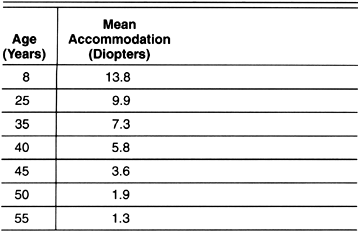Presbyopia is corrected by use of a plus lens to make up for the lost automatic focusing power of the lens. The plus lens may be used in several ways. Reading glasses have the near correction in the entire aperture of the glasses, making them fine for reading but blurred for distant objects. Half-glasses can be worn to abate this nuisance by leaving the top open and uncorrected for distance vision. Bifocals do the same but allow correction of other refractive errors. Trifocals correct for distance vision by the top segment, the middle distance by the middle section, and the near distance by the lower segment. Progressive power lenses similarly correct for far, middle, and near distances but by progressive change in lens power rather than stepped changes.

Myopia

When the image of distant objects focuses in front of the retina in the unaccommodated eye, the eye is myopic, or nearsighted (Figure 20-21). If the eye is longer than average, the error is called axial myopia. (For each additional millimeter of axial length, the eye is approximately 3 diopters more myopic.) If the refractive elements are more refractive than average, the error is called curvature myopia or refractive myopia. As the object is brought closer than 6 meters, the image moves closer to the retina and comes into sharper focus. The point reached where the image is most sharply focused on the retina is called the "far point." One may estimate the extent of myopia by calculating the reciprocal of the far point. Thus, a far point of 0.25 m would suggest a 4-diopter minus lens correction for distance. The myopic person has the advantage of being able to read at the far point without glasses even at the age of presbyopia. A high degree of myopia results in greater susceptibility to degenerative retinal changes, including retinal detachment.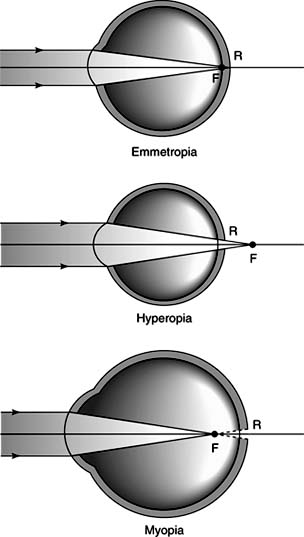Figure 20-21: Spherical refractive errors as determined by the position of the secondary focal point with respect to the retina.

Concave spherical (minus) lenses are used to correct the image in myopia. These lenses move the image back to the retina.

Hyperopia

Hyperopia (hypermetropia, farsightedness) is the state in which the unaccommodated eye would focus the image behind the retina (Figure 20-21). It may be due to reduced axial length (axial hyperopia), as occurs in certain congenital disorders, or reduced refractive error (refractive hyperopia), as exemplified by aphakia.

Hyperopia is a more difficult concept to explain than myopia. The term "farsighted" contributes to the difficulty, as does the prevalent misconception among laymen that presbyopia is farsightedness and that one who sees well far away is farsighted. If hyperopia is not too great, a young person may obtain a sharp distant image by accommodating, as a normal eye would to read. The young hyperopic person may also make a sharp near image by accommodating more-or much more than one without hyperopia. This extra effort may result in eye fatigue that is more severe for near work. The degree of hyperopia a person may have without symptoms is-like most clinical conditions-variable. However, the amount decreases with age as presbyopia (decrease in ability to accommodate) increases. Three diopters of hyperopia might be tolerated in a teenager but will require glasses later, even though the hyperopia has not increased. If the hyperopia is too high, the eye may be unable to correct the image by accommodation. The hyperopia that cannot be corrected by accommodation is termed manifest hyperopia. This is one of the causes of deprivation amblyopia in children and can be bilateral. There is a reflex correlation between accommodation and convergence of the two eyes. Hyperopia is therefore a frequent cause of esotropia (crossed eyes) and monocular amblyopia (see Chapter 12).

Latent Hyperopia

As explained above, a prepresbyopic person with hyperopia may obtain a clear retinal image by accommodation. The degree of hyperopia overcome by accommodation is known as latent hyperopia. It is detected by refraction after instillation of cycloplegic drops, which determines the sum of both manifest and latent hyperopia. Refraction with a cycloplegic is very important in young patients who complain of eyestrain when reading and is vital in esotropia, where full correction of hyperopia may achieve a cure.

Remember that a moderately "farsighted" person may see well for near or far when young. However, as presbyopia comes on, the hyperope first has trouble with close work-and at an earlier age than the nonhyperope. Finally, the hyperope has blurred vision for near and far and requires glasses for both near and far.

Astigmatism

In astigmatism, the eye produces an image with multiple focal points or lines. In regular astigmatism, there are two principal meridians, with constant power and orientation across the pupillary aperture, resulting in two focal lines. The astigmatism is then further defined according to the position of these focal lines with respect to the retina (Figure 20-22). When the principal meridians are at right angles and their axes lie within 20 degrees of the horizontal and vertical, the astigmatism is subdivided into astigmatism with the rule, in which the greater refractive power is in the vertical meridian; and astigmatism against the rule, in which the greater refractive power is in the horizontal meridian. Astigmatism with the rule is more commonly found in younger patients and astigmatism against the rule more commonly in older patients (Figure 20-23). Oblique astigmatism is regular astigmatism in which the principal meridians do not lie within 20 degrees of the horizontal and vertical. In irregular astigmatism, the power or orientation of the principal meridians changes across the pupillary aperture.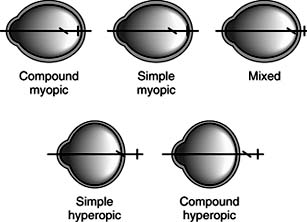Figure 20-22: Types of regular astigmatism as determined by the positions of the two focal lines with respect to the retina.Figure 20-23: Types of astigmatism as determined by the orientation of the principal meridians and the orientation of the correcting cylinder axis.

The usual cause of astigmatism is abnormalities of corneal shape. The crystalline lens may also contribute. In contact lens terminology, lenticular astigmatism is called residual astigmatism because it is not corrected by a spherical hard contact lens, which does correct corneal astigmatism.

Astigmatic errors can be corrected with cylindrical lenses, frequently in combination with spherical lenses. Because the brain is capable of adapting to the visual distortion of an uncorrected astigmatic error, new glasses that do correct the error may cause temporary disorientation, particularly an apparent slanting of images.

Natural History of Refractive Errors

Most babies are slightly hyperopic at birth. The hyperopia slowly decreases, with a slight acceleration in the teens, to approach emmetropia. The corneal curvature is much steeper (6.59 mm radius) at birth and flattens to nearly the adult curvature (7.71 mm) by about 1 year. The lens is much more spherical at birth and reaches adult conformation at about 6 years. The axial length is short at birth (17.3 mm), lengthens rapidly in the first 2 or 3 years (to 24.1 mm), then moderately (0.4 mm per year) until age 6, and then slowly (about 1 mm total) to stability at about 10 or 15 years. Presbyopia becomes manifest in the fifth decade.

Refractive errors are inherited. The mode of inheritance is complex, as it involves so many variables. Refractive error, though inherited, need not be present at birth any more than tallness, which is also inherited, need be present at birth. For example, a child who reaches emmetropia at age 10 years will probably soon become myopic. Myopia usually increases during the teens. This should be expected, just as the need for larger shoes is expected, and not looked on with alarm by the patient and parents. In a similar way, hyperopia usually decreases slightly during the teens. Myopia does not generally decrease with age, as is popularly believed, nor does farsightedness come with aging.

Anisometropia

Anisometropia is a difference in refractive error between the two eyes. It is a major cause of amblyopia because the eyes cannot accommodate independently and the more hyperopic eye is chronically blurred. Refractive correction of anisometropia is complicated by differences in size of the retinal images (aniseikonia) and oculomotor imbalance due to the different degree of prismatic power of the periphery of the two corrective lenses. Aniseikonia is predominantly a problem of monocular aphakia. Spectacle correction produces a difference in retinal image size of approximately 25%, which is rarely tolerable. Contact lens correction reduces the difference in image size to approximately 6%, which can be tolerated. Intraocular lenses produce a difference of less than 1%.

Correction of Refractive Errors

A. Spectacle Lenses:

Spectacles continue to be the safest method of refractive correction. To reduce nonchromatic aberrations, the lenses are made in meniscus form (corrected curves) and tilted forward (pantascopic tilt).

B. Contact Lenses:

The first contact lenses were glass fluid-filled scleral lenses. These were difficult to wear for extended periods and caused corneal edema and much ocular discomfort. Hard corneal lenses, made of polymethylmethacrylate, were the first really successful contact lenses and gained wide acceptance for cosmetic replacement of glasses. Subsequent developments include gas-permeable rigid lenses, made of cellulose acetate butyrate, silicone, or various silicone and plastic polymers, and soft contact lenses, made of various hydrogel plastics, all of which provide increased comfort but greater risk of serious complications.

Hard and gas-permeable lenses correct refractive errors by changing the curvature of the anterior surface of the eye. The total refractive power consists of the power induced by the back curvature of the lens, the base curve, together with the actual power of the lens due to the difference between its front and back curvatures. Only the second is dependent on the refractive index of the contact lens material. Hard and gas-permeable lenses overcome corneal astigmatism by modifying the anterior surface of the eye into a truly spherical shape.

Soft contact lenses, particularly the more flexible forms, adopt the shape of the patient's cornea. Thus, their refractive power resides only in the difference between their front and back curvature, and they correct little corneal astigmatism unless a cylindrical correction is incorporated to make a toric lens.

Contact lens base curves are selected according to corneal curvature, as determined by keratometry or trial fittings. The front curvature is then calculated from the results of overrefraction with a trial contact lens, or from the patient's spectacle refraction as corrected for the corneal plane.

Hard contact lenses are specifically indicated for the correction of irregular astigmatism, such as in keratoconus. Soft contact lenses are used for the treatment of corneal surface disorders, but for control of symptoms rather than for refractive reasons. All forms of contact lenses are used in the refractive correction of aphakia, particularly in overcoming the aniseikonia of monocular aphakia, and the correction of high myopia, in which they produce a much better visual image than spectacles. But the vast majority of contact lenses worn are for cosmetic correction of low refractive errors. This has important implications for the risks that can be reasonably accepted in the use of contact lenses. (Further discussion of therapeutic and cosmetic contact lens use, and the associated complications, is given in Chapter 6.)

C. Keratorefractive Surgery:

Keratorefractive surgery encompasses a range of methods for changing the curvature of the anterior surface of the eye. The expected refractive effect is generally derived from empirical results of similar procedures in other patients and not based upon mathematical optical calculations. Further discussion of the methods and outcome of keratorefractive procedures is included in Chapter 6.

D. Intraocular Lenses:

Implantation of an intraocular lens has become the preferred method of refractive correction for aphakia. A large number of designs are available, including rigid lenses, most commonly consisting of an optic made of polymethylmethacrylate and loops (haptics) made of the same material or polypropylene; and foldable lenses, made of silicone or hydrogel plastics, which can be inserted into the eye through a much smaller incision. The safest position for an intraocular lens is within an intact capsular bag following extracapsular surgery.

The most popular method of determining the necessary intraocular lens power is the empirical regression method of analyzing experience with lenses of one style in many patients, from which is derived a mathematical formula based on a constant for the particular lens (A), average keratometer readings (K), and axial length in millimeters (L). An example is the SRK (Sanders-Retzlaff-Kraff) equation: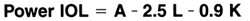This formula gives clinically satisfactory results in most cases. When the eye is not of average dimensions, such as in high myopia, the results are less good, and various modifications have been derived.

Certain theoretic formulas are also available, also utilizing a lens constant, keratometer readings, and axial length, together with estimated anterior chamber depth following surgery. Unfortunately, none of these formulas are based on trigonometric ray tracing methods, which do accurately predict the correct power of intraocular lens for an individual patient.

E. Clear Lens Extraction for Myopia:

Extraction of noncataractous lenses has been advocated for the refractive correction of myopia. For the process to have any success, the eye must be highly myopic, and the surgery is then sufficiently likely to have an adverse result that it is rarely justifiable.

METHODS OF REFRACTION

Determination of a patient's refractive correction can be achieved by objective or subjective means and is best accomplished by a combination of the two methods where possible.

Objective Refraction

Objective refraction is performed by retinoscopy, in which a streak of light, known as the intercept, is projected into the patient's eye to produce a similarly shaped reflex, the retinoscopic reflex, in the pupil (Figure 20-24). Parallel alignment of the intercept and the retinoscopic reflex indicates the presence of only a spherical error, or an additional cylindrical error in which the intercept coincides with one of the principal meridians. Rotation of the projected streak will determine which of these applies and the location of the other principal meridian in the case of a cylindrical error.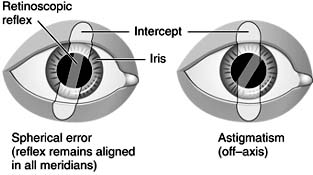Figure 20-24: The retinoscopic reflex.

The intercept is then swept across the patient's pupil, and the effect on the retinoscopic reflex is noted (Figure 20-25). If it moves in the same direction (with movement), plus lenses are placed before the patient's eye; and if it moves in the opposite direction (against movement), minus lenses are added-until the pupillary reflex fills the whole pupillary aperture and no movement is detected (point of neutralization). When the point of neutralization has been reached, the patient's refractive error has been corrected with an additional correction related to the distance between the patient and examiner (working distance). Spherical power equal to the reciprocal of the working distance (measured in meters) is subtracted to compensate for this additional correction and obtain the patient's refractive correction. The working distance is usually 2/3 m, and the correction to be subtracted for the working distance thus is usually 1.5 D.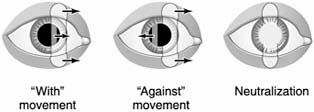Figure 20-25: Movement of the retinoscopic reflex.

Automated refractors are available to rapidly determine the objective refraction, but they are not useful in young children or in adults with significant anterior segment disease.

Subjective Refraction

In cooperative patients, subjective refraction produces more accurate results than objective refraction. It relies on the patient's response to alterations in lens power and orientation, using objective refraction or the patient's current refractive correction as the starting point.

The spherical correction is checked by small changes, initially increasing the plus power so as to overcome any accommodative effort, until the clearest image is obtained. The duochrome test of black letters on red and green backgrounds uses the normal chromatic aberration of the eye to refine spherical correction. When the black letters of the two halves of the chart are equally clear, the end point has been reached.

A cross cylinder consists of two planocylindrical lenses of equal power but opposite sign superimposed such that their axes of refractive power lie at right angles to one another. This is equivalent to a spherocylindrical lens in which the power of the cylinder is twice the power of the sphere and of the opposite sign. The cross cylinder allows rapid small changes in the axis and power of a cylindrical correction.

Cycloplegic Refraction

In the determination of full hyperopic refractive correction, either in the management of childhood esotropia or the assessment of eyestrain in adult hyperopes, it is necessary to overcome accommodation. This can usually be achieved in adults by fogging techniques in which plus lenses are used to overcome accommodative effort. But otherwise-and always in children-accommodation has to be relaxed by cycloplegic drugs. Cyclopentolate 1%, 1 drop instilled twice 30 minutes prior to refraction, may be sufficient, but atropine 0.5% or 1% ointment, applied twice a day for 3 days, may be necessary in children with dark irides and in the initial assessment of accommmodative esotropia. Parents should be warned of the symptoms of atropine toxicity (fever, flushed face, and rapid pulse) and the necessity for discontinuing treatment, cooling the child with sponge bathing, and-in severe cases-seeking urgent medical assistance.

List of Figures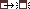Figure 20-1: Refraction of light as it enters a transparent medium of higher refractive index n′Figure 20-2: Example of the laws of reflection and refraction.Figure 20-3: Example of the critical angle.Figure 20-4: Illustration of three rays traced in trigonometric ray tracing.Figure 20-5: Algebraic thin lens approximations. All lengths in meters.Figure 20-6: Description of a thick lens in Gaussian optics.Figure 20-7: Ray tracing through plus and minus lenses.Figure 20-8: Chromatic aberration of lenses.Figure 20-9: Spherical aberration of a biconvex lens.Figure 20-10: A planocylindrical lens with axis in the horizontal meridian.Figure 20-11: Top: Illustration of prism base notation. Bottom: Illustration of cylinder axis notation.Figure 20-12: The conoid of Sturm, formed by light refracted by an astigmatic lens.Figure 20-13: Cross diagram and equivalent combinations, including longhand notations, for a spherocylindrical lens.Figure 20-14: Calibration of prisms. Glass prisms and spectacle prisms are calibrated according to the Prentice position, whereas plastic prisms are calibrated according to the position of minimum deviation.Figure 20-15: Example of the prism as used in ophthalmology.Figure 20-16: Power of a prism in prism diopters.Figure 20-17: The Fresnel prism.Figure 20-18: The reduced schematic eye.Figure 20-19: The optical system of the eye, illustrating the three-lens concept.Figure 20-20: Snellen block E.Figure 20-21: Spherical refractive errors as determined by the position of the secondary focal point with respect to the retina.Figure 20-22: Types of regular astigmatism as determined by the positions of the two focal lines with respect to the retina.Figure 20-23: Types of astigmatism as determined by the orientation of the principal meridians and the orientation of the correcting cylinder axis.Figure 20-24: The retinoscopic reflex.Figure 20-25: Movement of the retinoscopic reflex.

List of TablesTable 20-1: Indices of refraction and dispersion values of some substances of ophthalmologic interest.Table 20-2: Table of accommodation.10.1036/1535-8860.ch20